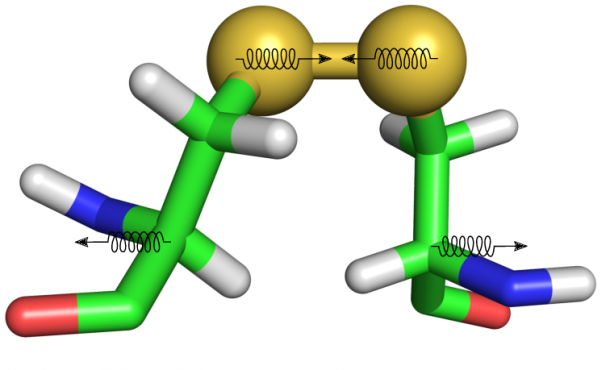# Tcl procedure to quantify pre-stress in S-S bonds

Here you will find a tcl script that contains a procedure able to calculate the properties of S-S bonds as defined by Schmidt et al. published in “Biochemistry (2006) Vol. 45 pp. 7429-7433.

The script identifies the S-S bonds in any protein considering that these information are supplied in the pdb file loaded in vmd. These information need to comply the format normally used in PDB files to described the presence of disulphide bonds.
This format is the following and examples can be found in any PDB file of proteins containing disulphide bonds:

## SSBOND 1 CYS A 3 CYS A 15

* The script calculates the dihedral angles chi1 to chi1-prime (see Schmidt et al. for details) and from those calculates the strain energy of the bond and the class (-RHSpiral,-LH/RH Staples, etc…) to which the bond belongs.

* Two output files are created:

– One file called SS-chis-summary_{PDBFILE}.dat that contains a summary of which atoms have been used to calculate the chi dihedrals, what are the atom indices etc…

– One file called SS-measures_{PDBFILE}.dat, which contains the values of the chi angles and other properties in the following order:

– Frame number. This is the number of the frame. For single PDB files (x-Ray structures) only one frame will be shown (column 1).
– χ1 to χ5 angle values in degrees (column 2:6).
– The strain energy of the bond (in that particular conformation) calculated in kJ/mol using the empirical formula proposed in the above-mentioned paper (column 7).
– The S-S distance in Å (column 8).
– The class of the S-S bond (column 9).

To use the script load vmd with the molecule (and trajectory) that you want to analyse
In the tcl/tk console window source the file typing “source \${SCRIPT}.tcl”
launch the procedure called ss typing ss \${FILE}.pdb that has been loaded when you launched VMD.